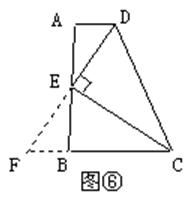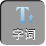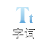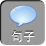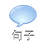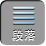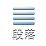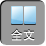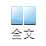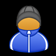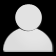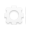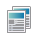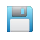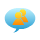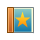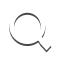-AA+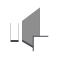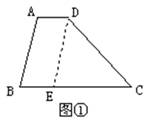∴四边形 ABED是平行四边形（两组对边分别平行的四边形是平行四边形）

DE= AB= 4 cmBE= AD= 2 cm

EC= BCBE= 72= 5 cm

1 cmCD9 cm

2、如图②，已知梯形 ABCD中， ADBC，∠ B=∠ C，求证：梯形 ABCD是等腰梯形．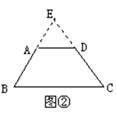EA= EDEB= EC（等角对等边）

AB= DC

∴梯形 ABCD是等腰梯形（两腰相等的梯形是等腰梯形）．

3、如图③，已知梯形 ABCD中， AD= 1.5 cmBC= 3.5 cm，对角线 ACBD，且 BD= 3 cmAC= 4 cm，求梯形 ABCD的面积．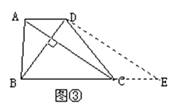∴四边形 ACED是平行四边形（两组对边分别平行的四边形是平行四边形）

CE= AD= 1.5 cmDE= AC= 4 cm

ACBD

DEBD

S梯形 ABCD=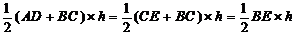h为梯形的高）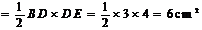4、如图④，已知梯形 ABCD中， DCABDAABADC= 1DA= 2AB= 3，求∠ B的度数．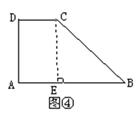DCABDAAB

∴四边形 AECD是矩形（有三个角是直角的四边形是矩形）

AE= DC= 1CE= DA= 2

AB= 3

EB= ABAE= 31= 2= CE

∴∠ B= 45°（等腰直角三角形锐角度数等于 45°）．

5、如图⑤，已知梯形 ABCD中， DCABAD= BC，延长 ABE，使 BE= CD，求证： AC= CE.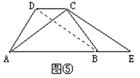∴梯形 ABCD是等腰梯形（两腰相等的梯形是等腰梯形）

AC= BD（等腰梯形两条对角线相等）

DCABDCBEBE= CD

∴四边形 DBEC是平行四边形（一组对边平行并且相等的四边形是平行四边形）

BD= CE（平行四边形对边相等）

AC= CE